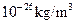Chapter 34, Problem 30PE

Chapter
Section
Textbook Problem

To get an idea of how empty deep spam is on the average, perform the following calculations:(a) Find the volume our Sun would occupy if it had an average density equal to the critical density ofthought necessary to halt the expansion of the universe.(b) Find the radius of a sphere of this volume in light years.(c) What would this radius be if the density were that of luminous matter, which is approximately 5% that of the critical density?(d) Compare the radius found in part (c) with me 4-ly average separation of stars in the aims of the Milky Way.

To determine

(a)

The volume occupied by Sun.

Explanation

Given:

Critical mass density, ρ=1026kg/m3

Mass of Sun, ms=1.989×1030kg

Formula:

Formula to calculate the volume occupied by Sun is,

ρ=msV   ......... (I)

Where,

• ms is mass of Sun.
• V is volume occupied by Sun.

Calculation:

Substitute 1026kg/m3 for ρ and 1.989×1030kg for ms in equation (I)

To determine

(b)

The radius of the sphere.

To determine

(c)

The radius of the sphere of the luminous matter.

To determine

(d)

The comparison of the radius of the luminous matter with 4 ly average separation of stars.

Still sussing out bartleby?

Check out a sample textbook solution.

See a sample solution

The Solution to Your Study Problems

Bartleby provides explanations to thousands of textbook problems written by our experts, many with advanced degrees!

Get Started